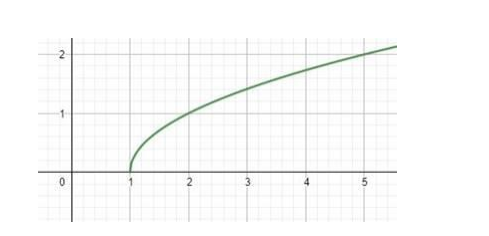# Write the domain and the range of the function, f(x) =

Question:

Write the domain and the range of the function, f(x) = $\sqrt{x-1}$

Solution:

The graph of f(x) is(i) Domain

Domain for $\sqrt{x}$ is $[0, \infty)$.

Hence, domain for $\sqrt{x-1}$ is $[1, \infty)$.

(ii) Range

As the range of function $f(x)=\sqrt{x}$ is given by the interval $[0,+\infty)$.

The graph of the given function $f(x)=\sqrt{x}-1$ is the graph of $\sqrt{x}$ shifted 1 unit to the right. A shift to the right does not affect the range.

Hence the range of $f(x)=\sqrt{x}-1$ is also given by the interval: $[0,+\infty)$.